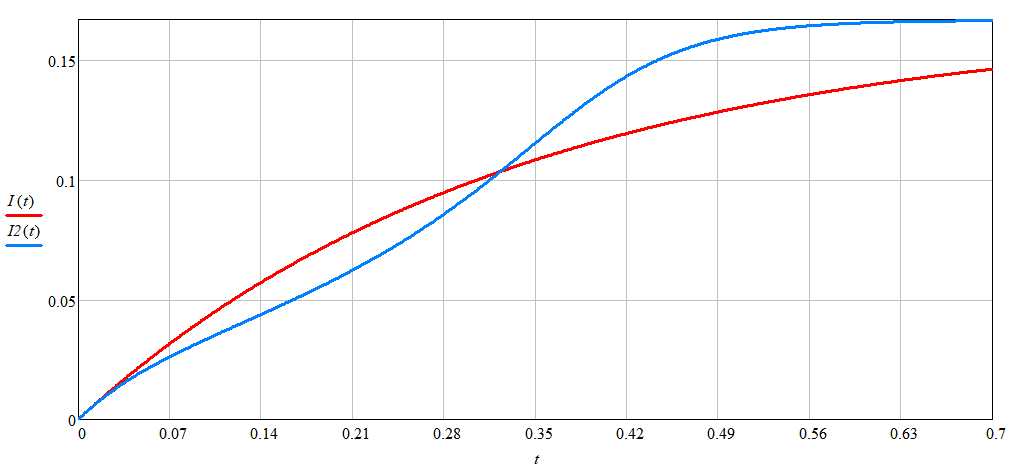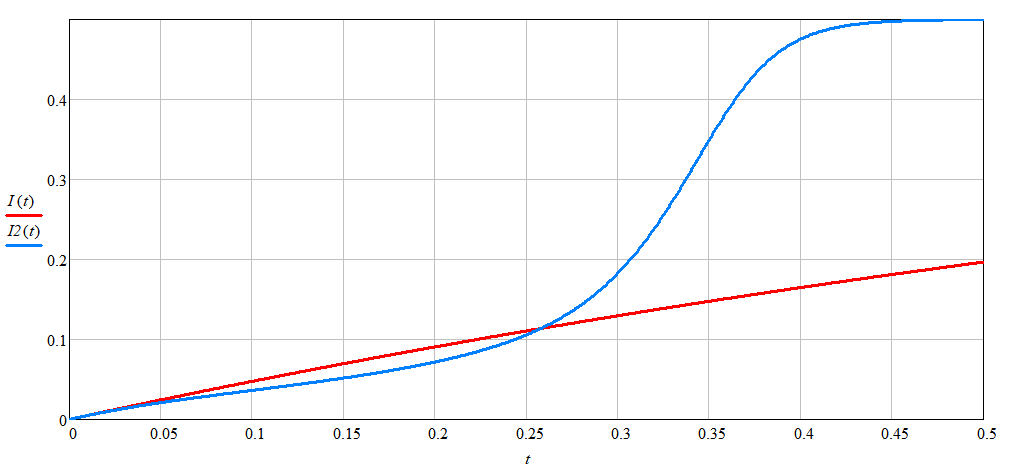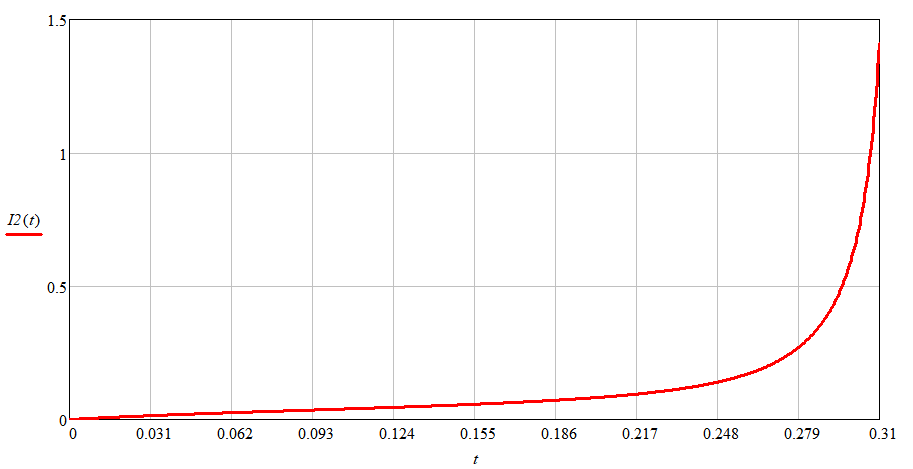Research website of Vyacheslav Gorchilin
2023-05-01
Analytical equation for current and energy in a coil according to the Stoletov curve
It is known that the Stoletov curve  quite accurately describes the behavior of ferromagnets in a magnetic field. In a more earlier work, the author suggested a way to represent such a curve as a simple polynomial, and also - a technique for finding the coefficients for this polynomial. With their help, you can completely restore the appearance of the Stoletov curve, so they are recommended to be indicated in the ferrite passport by their manufacturer.
One of the advantages of the presented polynomial is the analytical solutions of differential equations for the current flowing through a coil with a ferromagnetic core, and also - calculation of the energy stored in it. Of course, they work in some permissible areas, which will be necessarily stipulated. We will introduce you to one such solution in this paper.
Find the current first
Recall that the inductance of a coil $$L$$ with a ferromagnetic core can be represented as $L(I) = L_0\, M\!(I) \tag{1}$ Where $M\!(I) = {1 + k_{12} I^2 \over 1 + k_{22} I^2 + k_{23} I^3} \tag{2}$ Here: $$I$$ is the current flowing through the coil, the inductance of which varies depending on this current along the curve $$M\!(I)$$, which is a polynomial with coefficients $$k_{12}, k_{22}, k_{23}$$. When the current is zero ($$I=0$$), the coil has an initial inductance $$L_0$$, usually indicated in its passport.
When a voltage is applied to such a coil, the current $$I 003d I (t)$$ begins to grow in it, which can be found from the following differential equation: $L_0 {\partial [I\, M\!(I)] \over \partial t} + R\, I = U \tag{3}$ Here: $$t$$ is the time varying from zero to some finite value, and $$R$$ is the active resistance of the coil winding.
Let's see more clearly (Fig. 1.2) how the dependence of the current on time changes: when the coefficients at $$M\!(I)$$ are equal to zero (classical dependence), and when the coefficients at $$M\!(I)$$ are nonzero (real dependence). In these figures, the red graph is plotted for $$k_{12} = k_{22} = k_{23} = 0$$, and blue for $$k_{12} = 689, k_{22} = 179, k_{23} = 4321$$. Latest data removed from real ferrite.Fig.1. Coil current versus time at U=0.5, L0=1, R=3Fig.2. Dependences of the current in the coil on time at U=0.5, L0=1, R=1
One would expect that when R tends to zero, the current graph will become completely rectangular. In fact, this is not the case, which will be shown below (an example is Figures 3 and 4), and, under certain restrictions, this approach can be applied to any coils with a high quality factor.
In this paper, we will consider this idealized case, when the active resistance of the coil is zero, which means that equation (3) will be slightly simplified: $L_0 {\partial [I\, M\!(I)] \over \partial t} = U, \quad R=0 \tag{4}$ This means its solution in the form: $I = {U\, t \over L_0\, M\!(I)} \tag{5}$ The problem here is that this equation, although seemingly simple, is nevertheless transcendental, because it is impossible to find the current value by simple data substitution. Let's show this in more detail by expanding the polynomial: $I = {U\, t \over L_0}\, {1 + k_{22} I^2 + k_{23} I^3 \over 1 + k_{12} I^2} \tag{6}$ To obtain an analytical solution for searching for current, it is necessary to solve a cubic equation. This is what we will do below using the general solution method . The general solution would thus be the following: $I(t) = {1 \over 3 \left[ k_{12} - k_{23}\, i \right]} \left[k_{22}\, i + \sqrt{B + \sqrt{B^2 - 4 A^3} \over 2} + \sqrt{2 A^3 \over B + \sqrt{B^2 - 4 A^3} } \right] \tag{7}$ Please note that in the resulting expression, the spanning roots are of the third degree, and the simplifications introduced here are found as follows: $i = {U \over L_0} t \\ A = (k_{22}\, i)^2 + 3(k_{23}\, i - k_{12}) \\ B = 2 (k_{22}\, i)^3 + 9\, k_{22}\, i (k_{23}\, i - k_{12}) + 27\, i ( k_{23}\, i - k_{12})^2 \tag{8}$ Formula (7) is applicable under the following process time constraints: $t_{max} \lt {k_{12} \over k_{23}} {L_0 \over U} \tag{9}$
It is noteworthy that the obtained tmax can be considered as the saturation time of the coil core, provided that its quality factor is high. Read here for more on this.
Despite some limitations (9), we got quite an analytical dependence of the current in the coil on time (7.8).Fig.3. The dependence of the current in the coil on time, obtained by solving the diff. equations (4)Fig.4. The dependence of the current in the coil on time, obtained by formula (7)
Graphs 3 and 4 show the result obtained in comparison with the standard solution of the differential equation. Recall that we took the active resistance equal to zero (R=0), the polynomial coefficients are the same as for Figure 2, and the rest of the data here are: U=0.5, L0=1. In this case, the calculated value of the core saturation time is: tmax = 0.31. The routine for MathCAD is here.
Energy in an inductor
We can get the energy in the coil if formula (4) is multiplied on both sides by the current, after which we integrate this expression over time: $L_0 \int \limits_0^t I\, \partial [I\, M\!(I)] = \int \limits_0^t U\, I\, \partial t \tag{10}$ On the right in (10) the energy stored in the coil is expressed, and on the left - the energy spent on the appearance of current in it. Obviously, with zero active resistance of the coil winding, these energies are equal. Thus, the energy in the coil will be like this: $W_L = L_0 \int \limits_0^t I\, \partial [I\, M\!(I)] \tag{11}$ Using the method of integration by parts, we find the final solution: $W_L = L_0\, M\!(I)\, I^2 - L_0 \int \limits_0^{I} I\, M\!(I)\, \partial I \tag{12}$ Formula (12) allows you to find the potential energy in the coil, in which $$I$$ can be found from expression (7), if this current at the moment $$t$$ is not known. It is also equal to the energy expended by the power source on the appearance of current $$I$$ in the coil. Note that in contrast to the classical formula $$W_L = L I^2 / 2$$, here the nature of the dependence of the ferromagnet (coil core) on the current passing through it is taken into account, which introduces significant (sometimes by orders of magnitude) changes in this energy. Therefore, the formulas obtained here, in addition to theoretical calculations, can be used in the calculations of parametric generators or pulse circuits, in which a large load is placed on ferromagnetic cores.
Materials used
1. Wikipedia. Stoletov's Curve.
2. Wikipedia. Cubic equation.